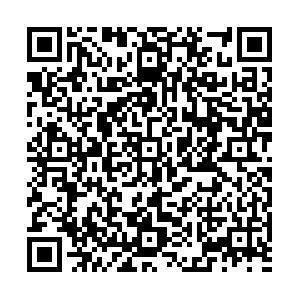# Strongly lensed gravitational waves as probes to test the cosmic distance duality relation

• The cosmic distance relation (DDR) associates the angular diameters distance ($D_A$) and luminosity distance ($D_L$) by a simple formula, i.e., $D_L = (1+z)^2D_A$. The strongly lensed gravitational waves (GWs) provide a unique way to measure $D_A$and $D_L$simultaneously to the GW source, hence they can be used as probes to test DDR. In this study, we investigated the use of strongly lensed GW events from the future Einstein Telescope to test DDR. We assumed the possible deviation of DDR as $(1+z)^2D_A/D_L = \eta(z)$, and considered two different parametrizations of $\eta(z)$, namely, $\eta_1(z) = 1+\eta_0 z$and $\eta_2(z) = 1+\eta_0 z/(1+z)$. Numerical simulations showed that, with about 100 strongly lensed GW events observed by ET, the parameter $\eta_0$was constrained at 1.3% and 3% levels for the first and second parametrizations, respectively.
••Get Citation
Hai-Nan Lin, Xin Li and Li Tang. Strongly lensed gravitational waves as the probes to test the cosmic distance duality relation[J]. Chinese Physics C. doi: 10.1088/1674-1137/abc53a
Hai-Nan Lin, Xin Li and Li Tang. Strongly lensed gravitational waves as the probes to test the cosmic distance duality relation[J]. Chinese Physics C.Milestone
Article Metric

Article Views(128)
Cited by(0)
Policy on re-use
To reuse of subscription content published by CPC, the users need to request permission from CPC, unless the content was published under an Open Access license which automatically permits that type of reuse.
###### 通讯作者: 陈斌, bchen63@163.com
• 1.

沈阳化工大学材料科学与工程学院 沈阳 110142

Title:
Email:

## Strongly lensed gravitational waves as probes to test the cosmic distance duality relation

###### Corresponding author: Li Tang, tang@cqu.edu.cn
• 1. Department of Physics, Chongqing University, Chongqing 401331, China
• 2. Department of Math and Physics, Mianyang Normal University, Mianyang 621000, China

Abstract: The cosmic distance relation (DDR) associates the angular diameters distance ($D_A$) and luminosity distance ($D_L$) by a simple formula, i.e., $D_L = (1+z)^2D_A$. The strongly lensed gravitational waves (GWs) provide a unique way to measure $D_A$and $D_L$simultaneously to the GW source, hence they can be used as probes to test DDR. In this study, we investigated the use of strongly lensed GW events from the future Einstein Telescope to test DDR. We assumed the possible deviation of DDR as $(1+z)^2D_A/D_L = \eta(z)$, and considered two different parametrizations of $\eta(z)$, namely, $\eta_1(z) = 1+\eta_0 z$and $\eta_2(z) = 1+\eta_0 z/(1+z)$. Numerical simulations showed that, with about 100 strongly lensed GW events observed by ET, the parameter $\eta_0$was constrained at 1.3% and 3% levels for the first and second parametrizations, respectively.

### HTML关注分享DownLoad:  Full-Size Img  PowerPoint